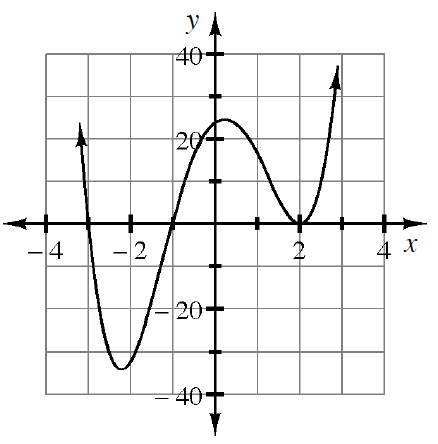### Home > PC3 > Chapter 4 > Lesson 4.1.2 > Problem4-27

4-27.Write a possible equation for the graph at right.

If $x=n$ is a root ($x$-intercept), then $(x−n)$ is a factor in the equation of the function.

1. Explain how you can decide if your equation represents the graph. What can you do to the equation to make it a better fit for the graph if needed?

$f(x)=a(x+3)(x+?)(x−?)^2$

2. The graph goes through the point $(1,16)$. Use this information to test the accuracy of your equation and make adjustments if needed.

Solve: $16=a(1+3)(1+?)(1−?)^2$